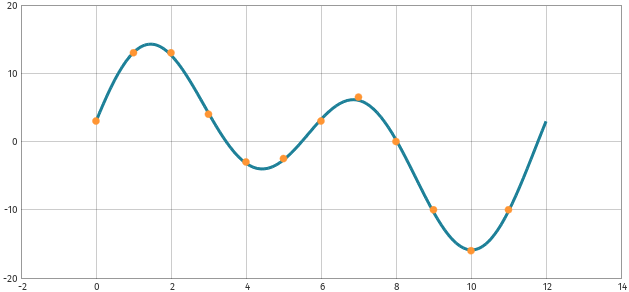# WORHP Kata of April 2023: Maximum Euclidean distance ⌚ 2023-04-01

A kata (in the context of programming) is a small training unit to practise coding. In this series of WORHP Katas we present standard problems of optimization which you can use to get familiar with optimization (and our optimization software WORHP).

Kata: We want to find the line $y=mx+t$ which minimizes the maximum Euclidean distance to the points $P(x_i,y_i), i=0,\dots,11$:
$\begin{array}{c|cccccccccccc} x_i & 0 & 1 & 2 & 3 & 4 & 5 & 6 & 7 & 8 & 9 & 10 & 11\\\hline y_i& 0 & 6 & 1 & 5 & 2 & 4 & 3 & 3 & 4 & 2 & 5 & 1\\ \end{array}$

Hint #1: There is a differentiable formulation which avoids the usage of max, if or similar.
Hint #2: You could also consider finding the line which minimizes the maximum distance only measured in the y-direction. However, with a little bit of school maths, you can compute the Euclidean distance between each point and the line.

A solution will be provided with the next WORHP Kata.

Solution of last WORHP Kata: For the given ordinate values $y_0=3, y_1=13, y_2=13, y_3=4, \dots$ we need some abscissa values, e.g.

$t_i = \frac{x_i}{12}\cdot 2\pi,\quad x_i=0,\dots, 11$

to solve this problem:
$\displaystyle\min\limits_{a,b,c,d} \sum_{i=1}^n \left(a \sin(t_i+b) + c \sin(2t_i+d) - y_i\right)^2$

Using this CPP code and our solver WORHP, we get after 12 iterations
$\begin{array}{rcl} a&=& 7.25421\\ b&=& -0.0198984\\ c&=& 9.7386\\ d&=& 0.322153, \end{array}$
which fits nicely to our data points: